# 04 - Logistic Regression¶

version 1.3, May 2018

# Review: Predicting a Continuous Response¶

In :
import pandas as pd
import zipfile
with zipfile.ZipFile('../datasets/glass.csv.zip', 'r') as z:
f = z.open('glass.csv')

Out:
ri na mg al si k ca ba fe glass_type
id
22 1.51966 14.77 3.75 0.29 72.02 0.03 9.00 0.0 0.00 1
185 1.51115 17.38 0.00 0.34 75.41 0.00 6.65 0.0 0.00 6
40 1.52213 14.21 3.82 0.47 71.77 0.11 9.57 0.0 0.00 1
39 1.52213 14.21 3.82 0.47 71.77 0.11 9.57 0.0 0.00 1
51 1.52320 13.72 3.72 0.51 71.75 0.09 10.06 0.0 0.16 1

Question: Pretend that we want to predict ri, and our only feature is al. How could we do it using machine learning?

Answer: We could frame it as a regression problem, and use a linear regression model with al as the only feature and ri as the response.

Question: How would we visualize this model?

Answer: Create a scatter plot with al on the x-axis and ri on the y-axis, and draw the line of best fit.

In :
%matplotlib inline
import matplotlib.pyplot as plt
plt.style.use('ggplot')

In :
# scatter plot using Pandas
glass.plot(kind='scatter', x='al', y='ri')

Out:
<matplotlib.axes._subplots.AxesSubplot at 0x7fcf7e2ffdd8>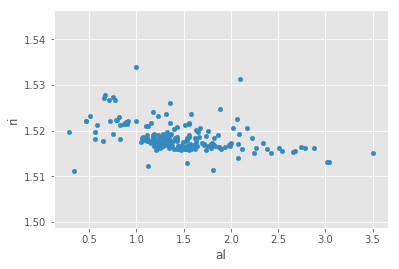In :
# equivalent scatter plot using Matplotlib
plt.scatter(glass.al, glass.ri)
plt.xlabel('al')
plt.ylabel('ri')

Out:
Text(0,0.5,'ri')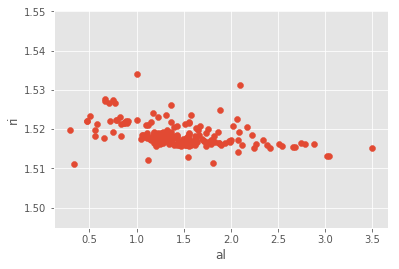In :
# fit a linear regression model
from sklearn.linear_model import LinearRegression
linreg = LinearRegression()
feature_cols = ['al']
X = glass[feature_cols]
y = glass.ri
linreg.fit(X, y)

Out:
LinearRegression(copy_X=True, fit_intercept=True, n_jobs=1, normalize=False)
In :
# make predictions for all values of X
glass['ri_pred'] = linreg.predict(X)

Out:
ri na mg al si k ca ba fe glass_type ri_pred
id
22 1.51966 14.77 3.75 0.29 72.02 0.03 9.00 0.0 0.00 1 1.521227
185 1.51115 17.38 0.00 0.34 75.41 0.00 6.65 0.0 0.00 6 1.521103
40 1.52213 14.21 3.82 0.47 71.77 0.11 9.57 0.0 0.00 1 1.520781
39 1.52213 14.21 3.82 0.47 71.77 0.11 9.57 0.0 0.00 1 1.520781
51 1.52320 13.72 3.72 0.51 71.75 0.09 10.06 0.0 0.16 1 1.520682
In :
# put the plots together
plt.scatter(glass.al, glass.ri)
plt.plot(glass.al, glass.ri_pred, color='red')
plt.xlabel('al')
plt.ylabel('ri')

Out:
Text(0,0.5,'ri')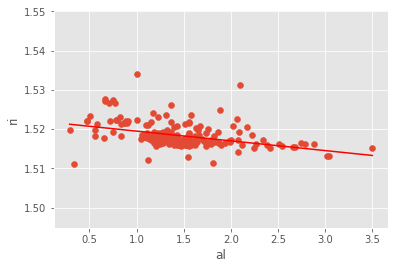### Refresher: interpreting linear regression coefficients¶

Linear regression equation: $y = \beta_0 + \beta_1x$

In :
# compute prediction for al=2 using the equation
linreg.intercept_ + linreg.coef_ * 2

Out:
array([1.51699012])
In :
# compute prediction for al=2 using the predict method
linreg.predict(2)

Out:
array([1.51699012])
In :
# examine coefficient for al
print(feature_cols, linreg.coef_)

['al'] [-0.00247761]


Interpretation: A 1 unit increase in 'al' is associated with a 0.0025 unit decrease in 'ri'.

In :
# increasing al by 1 (so that al=3) decreases ri by 0.0025
1.51699012 - 0.0024776063874696243

Out:
1.5145125136125304
In :
# compute prediction for al=3 using the predict method
linreg.predict(3)

Out:
array([1.51451251])

# Predicting a Categorical Response¶

In :
# examine glass_type
glass.glass_type.value_counts().sort_index()

Out:
1    70
2    76
3    17
5    13
6     9
7    29
Name: glass_type, dtype: int64
In :
# types 1, 2, 3 are window glass
# types 5, 6, 7 are household glass
glass['household'] = glass.glass_type.map({1:0, 2:0, 3:0, 5:1, 6:1, 7:1})

Out:
ri na mg al si k ca ba fe glass_type ri_pred household
id
22 1.51966 14.77 3.75 0.29 72.02 0.03 9.00 0.0 0.00 1 1.521227 0
185 1.51115 17.38 0.00 0.34 75.41 0.00 6.65 0.0 0.00 6 1.521103 1
40 1.52213 14.21 3.82 0.47 71.77 0.11 9.57 0.0 0.00 1 1.520781 0
39 1.52213 14.21 3.82 0.47 71.77 0.11 9.57 0.0 0.00 1 1.520781 0
51 1.52320 13.72 3.72 0.51 71.75 0.09 10.06 0.0 0.16 1 1.520682 0

Let's change our task, so that we're predicting household using al. Let's visualize the relationship to figure out how to do this:

In :
plt.scatter(glass.al, glass.household)
plt.xlabel('al')
plt.ylabel('household')

Out:
Text(0,0.5,'household')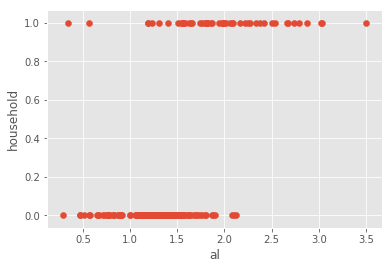Let's draw a regression line, like we did before:

In :
# fit a linear regression model and store the predictions
feature_cols = ['al']
X = glass[feature_cols]
y = glass.household
linreg.fit(X, y)
glass['household_pred'] = linreg.predict(X)

In :
# scatter plot that includes the regression line
plt.scatter(glass.al, glass.household)
plt.plot(glass.al, glass.household_pred, color='red')
plt.xlabel('al')
plt.ylabel('household')

Out:
Text(0,0.5,'household')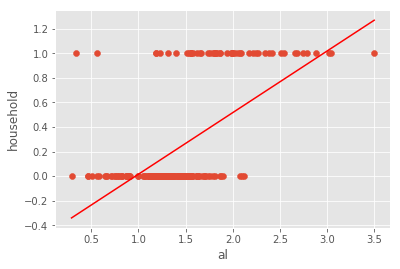If al=3, what class do we predict for household? 1

If al=1.5, what class do we predict for household? 0

We predict the 0 class for lower values of al, and the 1 class for higher values of al. What's our cutoff value? Around al=2, because that's where the linear regression line crosses the midpoint between predicting class 0 and class 1.

Therefore, we'll say that if household_pred >= 0.5, we predict a class of 1, else we predict a class of 0.

## $$h_\beta(x) = \beta_0 + \beta_1x_1 + \beta_2x_2 + ... + \beta_nx_n$$¶

• $h_\beta(x)$ is the response
• $\beta_0$ is the intercept
• $\beta_1$ is the coefficient for $x_1$ (the first feature)
• $\beta_n$ is the coefficient for $x_n$ (the nth feature)

### if $h_\beta(x)> 0.5$ then $\hat y = 1$¶

In :
# understanding np.where
import numpy as np
nums = np.array([5, 15, 8])

# np.where returns the first value if the condition is True, and the second value if the condition is False
np.where(nums > 10, 'big', 'small')

Out:
array(['small', 'big', 'small'], dtype='<U5')
In :
# transform household_pred to 1 or 0
glass['household_pred_class'] = np.where(glass.household_pred >= 0.5, 1, 0)

Out:
ri na mg al si k ca ba fe glass_type ri_pred household household_pred household_pred_class
id
22 1.51966 14.77 3.75 0.29 72.02 0.03 9.00 0.0 0.00 1 1.521227 0 -0.340495 0
185 1.51115 17.38 0.00 0.34 75.41 0.00 6.65 0.0 0.00 6 1.521103 1 -0.315436 0
40 1.52213 14.21 3.82 0.47 71.77 0.11 9.57 0.0 0.00 1 1.520781 0 -0.250283 0
39 1.52213 14.21 3.82 0.47 71.77 0.11 9.57 0.0 0.00 1 1.520781 0 -0.250283 0
51 1.52320 13.72 3.72 0.51 71.75 0.09 10.06 0.0 0.16 1 1.520682 0 -0.230236 0
In :
# plot the class predictions
plt.scatter(glass.al, glass.household)
plt.plot(glass.al, glass.household_pred_class, color='red')
plt.xlabel('al')
plt.ylabel('household')

Out:
Text(0,0.5,'household')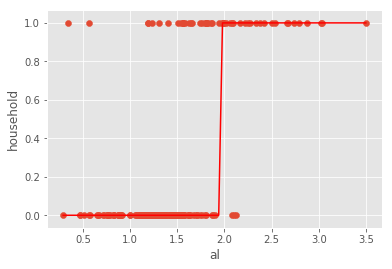$h_\beta(x)$ can be lower 0 or higher than 1, which is countra intuitive

Logistic regression can do what we just did:

In :
# fit a logistic regression model and store the class predictions
from sklearn.linear_model import LogisticRegression
logreg = LogisticRegression(C=1e9)
feature_cols = ['al']
X = glass[feature_cols]
y = glass.household
logreg.fit(X, y)
glass['household_pred_class'] = logreg.predict(X)

In :
# plot the class predictions
plt.scatter(glass.al, glass.household)
plt.plot(glass.al, glass.household_pred_class, color='red')
plt.xlabel('al')
plt.ylabel('household')

Out:
Text(0,0.5,'household')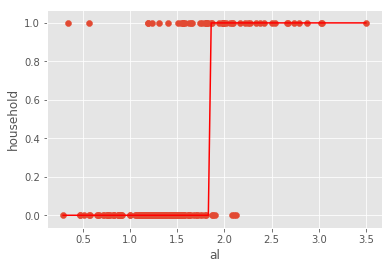What if we wanted the predicted probabilities instead of just the class predictions, to understand how confident we are in a given prediction?

In :
# store the predicted probabilites of class 1
glass['household_pred_prob'] = logreg.predict_proba(X)[:, 1]

In :
# plot the predicted probabilities
plt.scatter(glass.al, glass.household)
plt.plot(glass.al, glass.household_pred_prob, color='red')
plt.xlabel('al')
plt.ylabel('household')

Out:
Text(0,0.5,'household')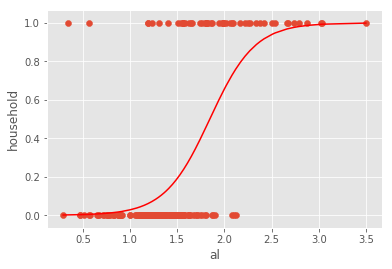In :
# examine some example predictions
print(logreg.predict_proba(1))
print(logreg.predict_proba(2))
print(logreg.predict_proba(3))

[[0.97161726 0.02838274]]
[[0.34361555 0.65638445]]
[[0.00794192 0.99205808]]


The first column indicates the predicted probability of class 0, and the second column indicates the predicted probability of class 1.

## Probability, odds, e, log, log-odds¶

$$probability = \frac {one\ outcome} {all\ outcomes}$$$$odds = \frac {one\ outcome} {all\ other\ outcomes}$$

Examples:

• Dice roll of 1: probability = 1/6, odds = 1/5
• Even dice roll: probability = 3/6, odds = 3/3 = 1
• Dice roll less than 5: probability = 4/6, odds = 4/2 = 2
$$odds = \frac {probability} {1 - probability}$$$$probability = \frac {odds} {1 + odds}$$
In :
# create a table of probability versus odds
table = pd.DataFrame({'probability':[0.1, 0.2, 0.25, 0.5, 0.6, 0.8, 0.9]})
table['odds'] = table.probability/(1 - table.probability)
table

Out:
probability odds
0 0.10 0.111111
1 0.20 0.250000
2 0.25 0.333333
3 0.50 1.000000
4 0.60 1.500000
5 0.80 4.000000
6 0.90 9.000000

What is e? It is the base rate of growth shared by all continually growing processes:

In :
# exponential function: e^1
np.exp(1)

Out:
2.718281828459045

What is a (natural) log? It gives you the time needed to reach a certain level of growth:

In :
# time needed to grow 1 unit to 2.718 units
np.log(2.718)

Out:
0.999896315728952

It is also the inverse of the exponential function:

In :
np.log(np.exp(5))

Out:
5.0
In :
# add log-odds to the table
table['logodds'] = np.log(table.odds)
table

Out:
probability odds logodds
0 0.10 0.111111 -2.197225
1 0.20 0.250000 -1.386294
2 0.25 0.333333 -1.098612
3 0.50 1.000000 0.000000
4 0.60 1.500000 0.405465
5 0.80 4.000000 1.386294
6 0.90 9.000000 2.197225

## What is Logistic Regression?¶

Linear regression: continuous response is modeled as a linear combination of the features:

$$y = \beta_0 + \beta_1x$$

Logistic regression: log-odds of a categorical response being "true" (1) is modeled as a linear combination of the features:

$$\log \left({p\over 1-p}\right) = \beta_0 + \beta_1x$$

This is called the logit function.

Probability is sometimes written as pi:

$$\log \left({\pi\over 1-\pi}\right) = \beta_0 + \beta_1x$$

The equation can be rearranged into the logistic function:

$$\pi = \frac{e^{\beta_0 + \beta_1x}} {1 + e^{\beta_0 + \beta_1x}}$$

In other words:

• Logistic regression outputs the probabilities of a specific class
• Those probabilities can be converted into class predictions

The logistic function has some nice properties:

• Takes on an "s" shape
• Output is bounded by 0 and 1

We have covered how this works for binary classification problems (two response classes). But what about multi-class classification problems (more than two response classes)?

• Most common solution for classification models is "one-vs-all" (also known as "one-vs-rest"): decompose the problem into multiple binary classification problems
• Multinomial logistic regression can solve this as a single problem

## Part 6: Interpreting Logistic Regression Coefficients¶

In :
# plot the predicted probabilities again
plt.scatter(glass.al, glass.household)
plt.plot(glass.al, glass.household_pred_prob, color='red')
plt.xlabel('al')
plt.ylabel('household')

Out:
Text(0,0.5,'household')In :
# compute predicted log-odds for al=2 using the equation
logodds = logreg.intercept_ + logreg.coef_ * 2
logodds

Out:
array([0.64722323])
In :
# convert log-odds to odds
odds = np.exp(logodds)
odds

Out:
array([1.91022919])
In :
# convert odds to probability
prob = odds/(1 + odds)
prob

Out:
array([0.65638445])
In :
# compute predicted probability for al=2 using the predict_proba method
logreg.predict_proba(2)[:, 1]

Out:
array([0.65638445])
In :
# examine the coefficient for al
feature_cols, logreg.coef_

Out:
(['al'], array([4.18040386]))

Interpretation: A 1 unit increase in 'al' is associated with a 4.18 unit increase in the log-odds of 'household'.

In :
# increasing al by 1 (so that al=3) increases the log-odds by 4.18
logodds = 0.64722323 + 4.1804038614510901
odds = np.exp(logodds)
prob = odds/(1 + odds)
prob

Out:
0.9920580839167457
In :
# compute predicted probability for al=3 using the predict_proba method
logreg.predict_proba(3)[:, 1]

Out:
array([0.99205808])

Bottom line: Positive coefficients increase the log-odds of the response (and thus increase the probability), and negative coefficients decrease the log-odds of the response (and thus decrease the probability).

In :
# examine the intercept
logreg.intercept_

Out:
array([-7.71358449])

Interpretation: For an 'al' value of 0, the log-odds of 'household' is -7.71.

In :
# convert log-odds to probability
logodds = logreg.intercept_
odds = np.exp(logodds)
prob = odds/(1 + odds)
prob

Out:
array([0.00044652])

That makes sense from the plot above, because the probability of household=1 should be very low for such a low 'al' value.Changing the $\beta_0$ value shifts the curve horizontally, whereas changing the $\beta_1$ value changes the slope of the curve.